# COVID-19 SIR Modelling for West Bengal, India

June 12, 2020

By  Aisik De

###### Move quickly from one page to another within COVID-19 Series from MATLAB Helper: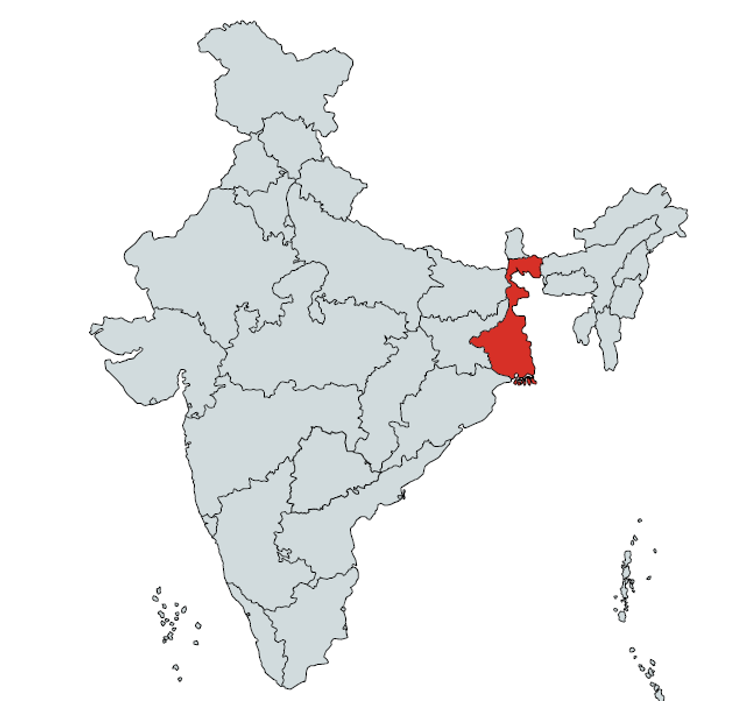Now we are going to show the pandemic analysis of another state of India, which is West Bengal (WB).

Every problem has many alternative solutions, so as our SIR modelling codes too. Now we have come up with another code to see the analysis and prediction done by it. By going through different approaches, we come up with different tactics to solve, so there is no correct solution, and none is wrong. It gives us an insight into various viewpoints as a solution to a problem can have.

Now let us do a deep understanding of our next code

### Section: Data Fetching

In this section, we will learn to know how to extract data from the URL as an input to our code

%% Data fetching
url="https://api.covid19india.org/csv/latest/state_wise_daily.csv";
date=datetime(record.Date,'inputformat','DD-MM-YYYY');

For this mathematical model, we have taken raw data of the Coronavirus stats from http://api.covid19india.org/csv/latest/state_wise_daily.csv.  The data from this URL has the stats for all the Indian states dated from the 14th of March 2020, which has the column-wise stats on 'confirmed,' 'recovered,' and 'deceased' of all the dates. Here we go on to a particular state, namely West Bengal, for a better understanding of the model.

We have saved our data in the variable called 'record.' The 'record=webread(url)' command reads content from the web service specified by URL and returns the content in 'url.' The function of webread is to read content from the RESTful web service. The web service provides a RESTful that returns data formatted as an internet media type such as JSON, XML, image, or text. The given URL is read, and the date is extracted by using datetime command in the format of 'DD-MM-YYYY.'

### Section: Data processing

This is the section where data extracted from the URL is fed for processing.

%% Data processing
[row,~]=size(record);
r=1;
for i=1:3
for index=row:-3:2
D_R_C(r,i)=record.WB(index);
r=r+1;
end
row=row-1;
r=1;
end

%totActive= sum(D_R_C(:,3))-sum(( D_R_C(:,1))+ sum(D_R_C(:,2)));
totalcases=cumsum(D_R_C(:,3));
totalrecovered=cumsum(D_R_C(:,2));
totaldeaths=cumsum(D_R_C(:,1));
totalactive=totalcases(end)-(totalrecovered(end)+ totaldeaths(end));

We go on by defining the array named as a row whose size is the same as that of the variable record, which we have defined previously. In this variable, all the data of West Bengal is stored. After this, we go on defining a variable called 'r' which is assigned a value 1.

Then we define a variable 'i' and 'index,' which are the loop controls. For the variable 'i,' the limits are defined from 1 and going to 3, and for the 'index,' the limits are from row to 2 with an interval of -3.

The body of the loop has D_R_C, which is a 3X3 matrix where D stands for the deceased, R stands for recovered, and C stands for confirmed. So, according to it, all the stats of the deceased are stored in D, and the same applies to all other variables that are R and C according to each date.

By now, we have reached the end of our second for loop with the decrease in the value of row and then the end of first for loop too. Now we have created an array of confirmed and recovered stats that will contain the infected and recovered cumulative data, respectively. Our purpose behind taking the data cumulative is because the data provided to us is on daily analysis, so we need to add it to the previous data, and hence we get the total number of active cases.

### Section: Conclusion and Determining the Susceptible

Now moving on further we define the number of susceptibles

%% Conclusion and Determining the Susceptible
total_pop=102741588;
percentage_affected=(totalcases(end)/total_pop)*100;
fprintf("Affected percentage of total population is %d \n",...
percentage_affected);
sus_percentage=percentage_affected*30;
sus=((total_pop-totalcases(end))/100)*sus_percentage;
fprintf("Considering susceptible as 30 x Current affected people: %d\n",...
sus);
sus_c=total_pop*10/100;

We have defined a variable 'total_pop,' which is assigned with the value of the total population of West Bengal. With the 'percentage_affected' variable, we will be able to calculate the population, which was affected by taking the end value of the array of 'totalcases.'

In 'totalcases,' the total confirmed case of each date starting from 1st day is stored, so the last date, i.e., 'totalcases(end),' will give the recent total confirmed case. Now, this information is used to calculate the percentage of affected people out of the total cases and the total. After the calculation, the percentage affected on the last day of analysis is shown.

To find the percentage of the susceptible population, we have taken a variable 'sus_percentage,' which stores the number of susceptible by taking the affected percentage thirty times. After the calculation of the susceptible, it is stored in the variable called 'sus,' and its value is displayed.

Here we have also taken an assumption that a certain percentage of the population is not immune to the virus which we have defined by the variable 'Sus_c' whose value is defined as 10 percent of the total population

### Section: Calculation transmission rate

Now we will analyse about the transmission rate of the virus.

%% Calculation new transmission rate
for u=1:1:size(D_R_C(:,3))
factor(u)=D_R_C(u,3)/sus_c;
sus_c=sus_c-D_R_C(u,3);

end
avg=sum(factor)/length(factor);

We started with the for loop in which the variable 'u' is driven from 1 to the length of D_R_C  third column, all row. Inside D_R_C(:,3), we have stored the confirmed case of that particular day.

Then we have another variable 'factor()' which stores the number of people who are infected every day with this virus. We are calculating this by dividing the value of each row with sus_c(10 percent of the total population. This marks the end of our loop, and then we head towards finding the average of the factor. The average factor is calculated, which is later assigned to the transmission ratio.

### Section: Calculation recovery rate

Now we will be calculating the recovery rate of the population that was infected.

%% Calculation of new recovery rate
for count1=1:length(D_R_C(:,2))
suum2(count1)=(D_R_C(i,2)/(totalcases(count1)-totaldeaths(count1)));
end

We start with the for loop again to find the size of the column for the recovered ones in the column D_R_C, and this is evaluated as the ratio of recovery on each day divided by totalcase - totaldeath, which gives as total active cases. So, we have the factor of recovery each day.

### Section: Time Calculation

In this section, the desired date of analysis is given as input. It is taken as input, and accordingly, the time is selected.

%% Time calculation
tmax=input("For how many days:");
%tmax=874;
minus=0;
while(1)
day=tmax+minus;
year=0;
month=0;
t=datetime;
if (day/365>1)
year=int16(day/365);
day=day-365*year;
end
if(day/30>1)
month=int16(day/30);
day=day-30*month;
end
f=yyyymmdd(t);
current_year=int16(f/10000);
res1=int16(mod(f,1000));
current_month=int16(res1/100);
res2=int16(mod(res1,100));
current_day=res2;
last_year=current_year+year;
lastmonth=current_month+month;
last_day=current_day+day;
d1=datetime(last_year,lastmonth,last_day);
range_day=t:d1;
if(tmax==length(range_day))
break;
else
minus=tmax-length(range_day);
end
end

### Section: Modelling the equation

In this section we will be modelling the equation which we have formed above for the calculation of various parameters

%% Modelling the Equation
transmission_ratio=avg;
recover_ratio=sum(suum2)/length(D_R_C(:,2));
t=1:1:tmax;
Nt=length(t);
I=zeros(1,tmax);
S=zeros(1,tmax);
R=zeros(1,tmax);
a=transmission_ratio;
b=recover_ratio;
I(1)=totalactive;
S(1)=sus;
for it=1:Nt-1
if(S(it)+I(it)+R(it)>=total_pop )
fprintf("here %d",it);
break;
end
ds(it)=-1*a*S(it)*I(it);
S(it+1)=S(it)+ds(it);
dI(it)=a*S(it)*I(it)-b*I(it);
I(it+1)=I(it)+dI(it);
dR(it)=b*I(it);
R(it+1)=R(it)+dR(it);
end

We start the modelling of our SIR model by assigning the value of 'transmision_ratio' and 'recover_ratio' as 'avg 'and the ratio of the sum to length, respectively.  Then we set the range for our time t with the interval of 1 day, and this value is stored in 'Nt.'

Then we initialise a vector 'I' indicating the infected population. We have used the 'zeros' function here which is used to create an array of all zeros and the command' I=zeros(1,tmax);' can be understood by an example as

• B=zeros(n) this statement returns an n-by-n matrix of zeros. An error message appears if n is not a scalar.

So here 'I=zeros(1,tmax);' returns a 1-by-tmax matrix of zeros and similar function happens for the other variables' S' and 'R'.

We now assign the value of transmission ratio, recovered ratio, and 'totalactive' cases to the variable 'a,' 'b,' and 'I' respectively. We also assign the value of susceptible 'sus' as 'S.'

Then we go onto start with a for loop for the variable 'it' with the limits 1 to Nt-1. We then put an If condition to check if the sum of S, I, R is below the total population or not. The sum of S, I, R cannot be more than the total population!

Now we come to the differential equations as:

• ds/dt formula is written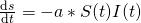• The S of next index is updated with ds,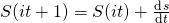• The di/dt formula is written as,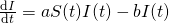Then our ‘I‘ is updated to the value of ‘S’ and the value of R is updated later on before that: -

dr/dt is written as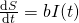### Section: Plotting

Now its time to see what we obtain as a result of our SIR modelling.

%% Plotting
plot(range_day,S,'y');
hold on;
plot(range_day,I,'R');
hold on;
plot(range_day,R,'g');
legend("Susceptible","Infected","Recovered","Location","best");

We have a plot for susceptible, infected, and recovered on the y-axis and the time denoted in the x-axis.

### Section: Result

In this section, we will obtain the result of our plotting.

%% Result
SUS_end=double.empty(tmax,0);
INFE_end=double.empty(tmax,0);
SUS_end=range_day(1==double(30>round(S)));
INFE_end=range_day(1==double(30>round(I)));
if(length(INFE_end)>1)
fprintf("on %s All infected will be recovered\n",INFE_end(1));
end
if(length(SUS_end)>1)
fprintf("on %s All Susceptible will be gone\n",SUS_end(1));
end

The value of any index if below 30 is displayed as the end of that case on the day of the same index. The code in current approach is expecting COVID-19 to end around 17th September 2020 for West Bengal. However we know that the pandemic is still increasing. Please revise the parameters to properly use current data and enhanced analysis.The different analysis leads to different conclusions, so does our modelling approaches too. We have new blogs covering the unique approach to analyse the SIR Model for the state of Odisha and a nation Colombia.

Conclusion:

We have used data untill 31st May 2020 for all these analysis. We have seen some approaches to modelling diseases such as COVID-19 through MATLAB. We have found essential characteristics within this process. We have done Data Analysis and Data Extraction and included a reliable source for the COVID-19 data. We have used preprocessing to extract a subset of the collected data and organise the data accurately. We have done Data Modelling with model construction, i.e. finding the best model that fits the COVID-19 pandemic. We have done the calculation of model parameters using real collected data and finally done the Data Visualisation. All these steps were essential, and luckily, MATLAB has so many functions and tool to use in the given scenario.

Now summing our analysis and coming to a definite conclusion, India's COVID-19 case tally climbed from 100 to one lakh in just 64 days as per the data sourced from the Union Ministry of Health & Family Welfare (MoHFW). On comparing this with global data, sourced from Worldometers, it has been found out that India's COVID-19 growth rate is more than double the number of days as compared to Colombia.

### Important Note

Our COVID-19 codes and analysis have been made for educational and research purpose. We have shown different approaches of Pandemic Modelling for each state and the accuracy of result is not guaranteed for real-life situation. We wish for early end of this pandemic. Now it's in our hands; we need to take our responsibilities & take proper precautions.

Now let's have a quick view of the precautions.

#### Stay Home for non-essential activities!Avoid going to crowded areas. Work From Home and Be A Super Hero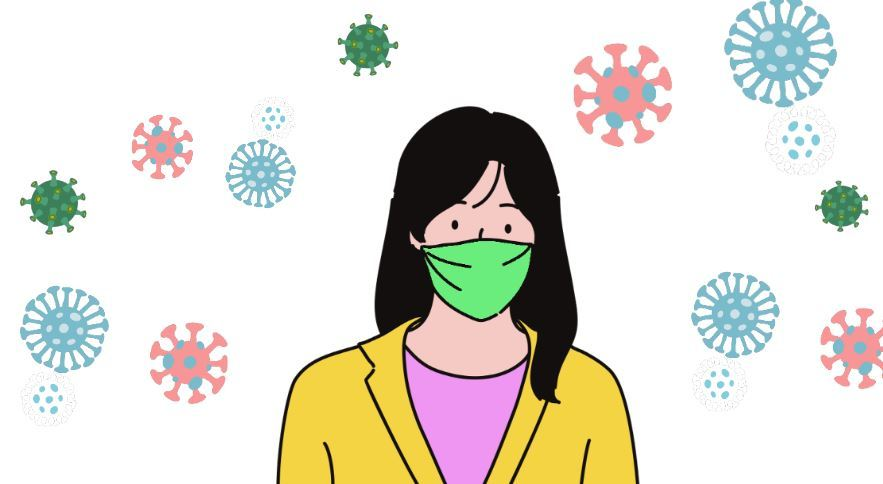Maintain distance while conversing with someone. Avoid touching your face frequently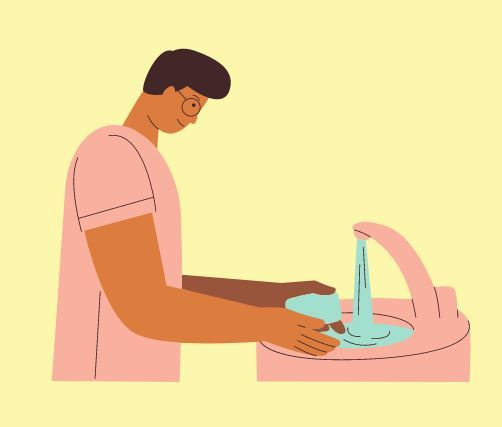Wash your hand for 20 seconds.  KEEP A SANTISER ALWAYS with you, wherever you go.While sneezing or coughing, always cover your mouth with a handkerchief or with your arm.

#### Home Quarantine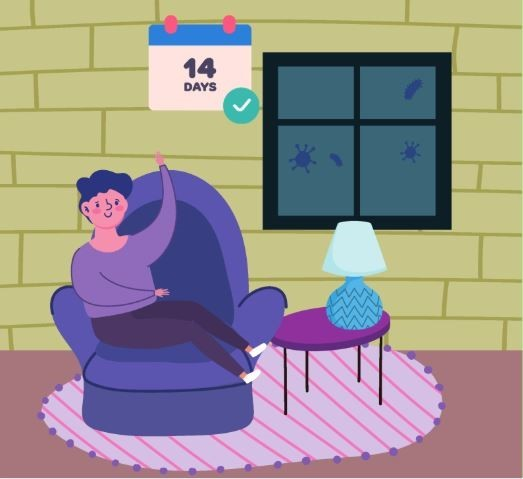If you have been facing the symptoms of Cold and Cough or the one's shown with COVID-19, then self-isolate yourself with home quarantine for 14 days.  So stay home stay safe; this is the best way you can contribute to the betterment of the nation.

The Blog has been created by Aisik De along with contributions or modifications from Nikita Mahoviya.

#### Aisik De

Tech freak with a zeal to learn and work on technologies. Acquired degree in Mechatronics , specialized in control system and power electronics.

•Nikita Mahoviya says:

The code is beautifully structured, and an explanation of every keyword made it easier to understand.

#### MATLAB Helper ®

Use Website Chat or WhatsApp at +91-8104622179